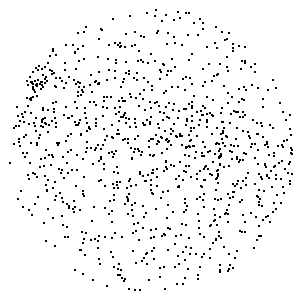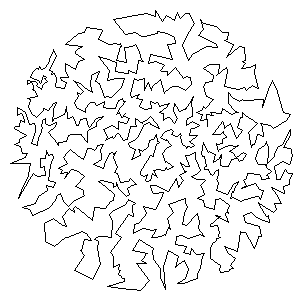COS 126 Traveling Salesperson Problem Programming Assignment

Given a set of N points in the plane, the goal of a traveling salesperson is to visit all of them (and arrive back home) while keeping the total distance traveled as short as possible. Write a program to compute an approximate solution to the traveling salesperson problem (TSP), and use it to find the shortest tour that you can, connecting a given set of points in the plane.Perspective. The importance of the TSP does not arise from an overwhelming demand of salespeople to minimize their travel distance, but rather from a wealth of other applications, many of which seem to have nothing to do with the TSP at first glance. Real world application areas include: vehicle routing, circuit board drilling, VLSI design, robot control, X-ray crystallography, machine scheduling, and computational biology.

Greedy heuristics. The traveling salesperson problem is a notoriously difficult combinatorial optimization problem, In principle, one can enumerate all possible tours, but, in practice, the number of tours is so staggeringly large (roughly N factorial) that this approach is useless. For large N, no one knows an efficient method that can find the shortest possible tour for any given set of points. However, many methods have been studied that seem to work well in practice, even though they are not guaranteed to produce the best possible tour. Such methods are called heuristics. Your main task is to implement the nearest neighbor and smallest increase insertion heuristics for building a tour incrementally. Start with a one-point tour (from the first point back to itself), and iterate the following process until there are no points left.

• Nearest neighbor heuristic:  Read in the next point, and add it to the current tour after the point to which it is closest. (If there is more than one point to which it is closest, insert it after the first such point you discover.)

• Smallest increase heuristic:  Read in the next point, and add it to the current tour after the point where it results in the least possible increase in the tour length. (If there is more than one point, insert it after the first such point you discover.)

Point data type. You are given a Point data type that represents a point in the plane. Each Point object can print itself to standard output, plot itself using standard draw, plot a line segment from itself to another point, and calculate the Euclidean distance between itself and another point. The Point class has the following API:

```public class Point (2D point data type)
---------------------------------------------------------------------------------------
Point(double x, double y)    // create the point (x, y)
String toString()                   // return string representation
void draw()                       // draw point using standard draw
void drawTo(Point b)              // draw line segment between the two points
double distanceTo(Point b)          // return Euclidean distance between the two points
```
In the tsp directory,, there is a class named Point which matches the required API.

Tour data type. Next, create a Tour data type that represents the sequence of points visited in a TSP tour. Represent the tour as a circular linked list of nodes, one for each point. Each Node will contain a Point and a reference to the next Node in the tour. Within Tour.java, define a nested class Node in the standard way.

```private class Node {
private Point p;
private Node next;

public Node(Point p) {  // create one Node
this.p = p;
this.next = null;
}
}```
Each Tour object should be able to print its constituent points to standard output, plot its points using standard draw, compute its total distance, and insert a new point using either of the two heuristics. Write a class named Tour that has the following API:
```public class Tour (TSP tour data type)
---------------------------------------------------------------------------------------
Tour()                                   // create an empty tour
Tour(Point a, Point b, Point c, Point d) // create a 4 point tour for debugging
void show()                                   // print the tour to standard output
void draw()                                   // draw the tour
double distance()                               // return the total distance of the tour
void insertSmallest(Point p)                  // insert p using smallest insertion heuristic
void insertNearest(Point p)                   // insert p using nearest neighbor heuristic
```

Input format. The input format will begin with two integers w and h, followed by pairs of real-valued x and y coordinates. All x coordinates will be real numbers between 0 and w; all y coordinates will be real numbers between 0 and h. As an example, the file tsp4.txt contains the following data

```600 600
532.6531 247.7551
93.0612 393.6735
565.5102 590.0000
10.0000  10.0000
```

Testing. Many test data files are also available. Once you implement Point and Tour, use the client program NearestInsertion.java to run the nearest insertion heuristic and print the resulting tour and its distance to standard output. Program SmallestInsertion.java is analogous but runs the smallest insertion heuristic. Programs NearestInsertionDraw.java and SmallestInsertionDraw.java are similar but they also plot the results using StdDraw. The programs read data from standard input. So, you should invoke as follows.

```% java NearestInsertion < tsp10.txt
```

Analysis.  Estimate the running time of your program as a function of N. Using TSPTimer, run the heuristics for increasing N as long as the execution time is less than 100 seconds. (i.e., Start with N = 1000, and repeatedly double N until the execution time is over 100 seconds.)

What to submit. Submit the files readme.txt, and Tour.java.

Contest and extra credit. Implement a better heuristic. For example, observe that any tour with paths that cross can be transformed into a shorter one with no crossing paths: add that improvement to your program. Here are some other ideas. We will award a special prize to whomever finds the shortest tour around the 1000-point set. Sorry. No partnering on the extra credit.

Submit a program ExtraCredit.java along with any accompanying files. Remember to include results and running instructions in your readme.

This assignment was developed by Bob Sedgewick and Kevin Wayne.